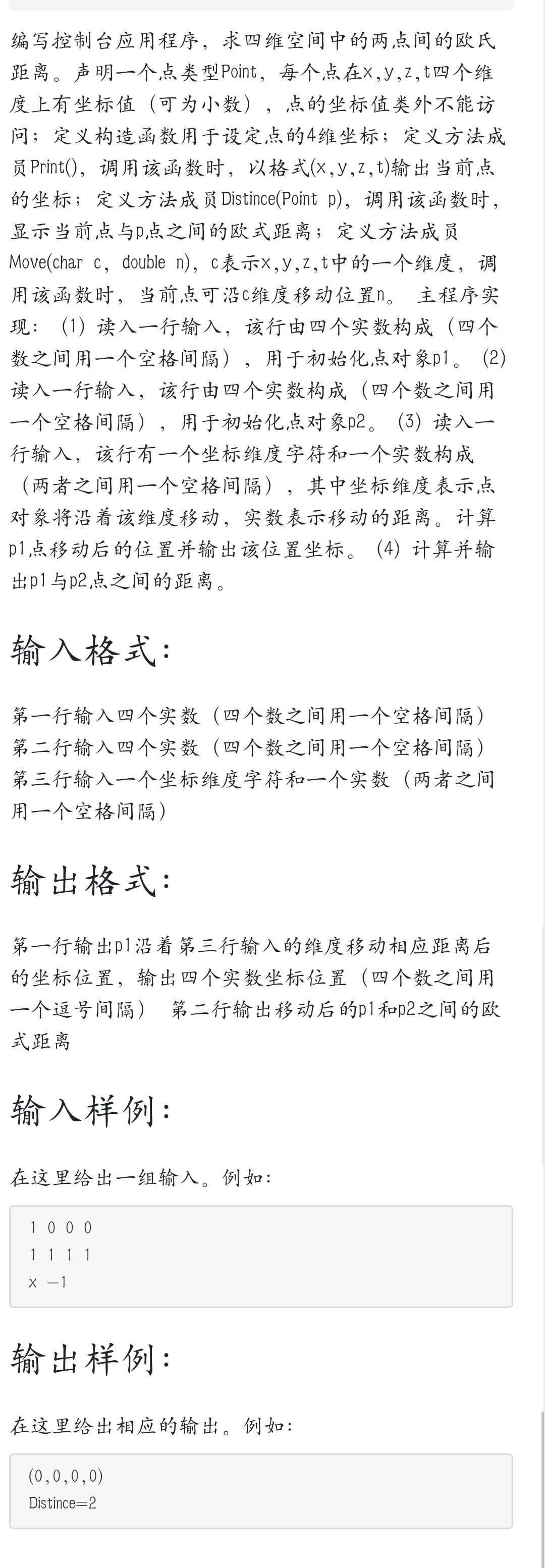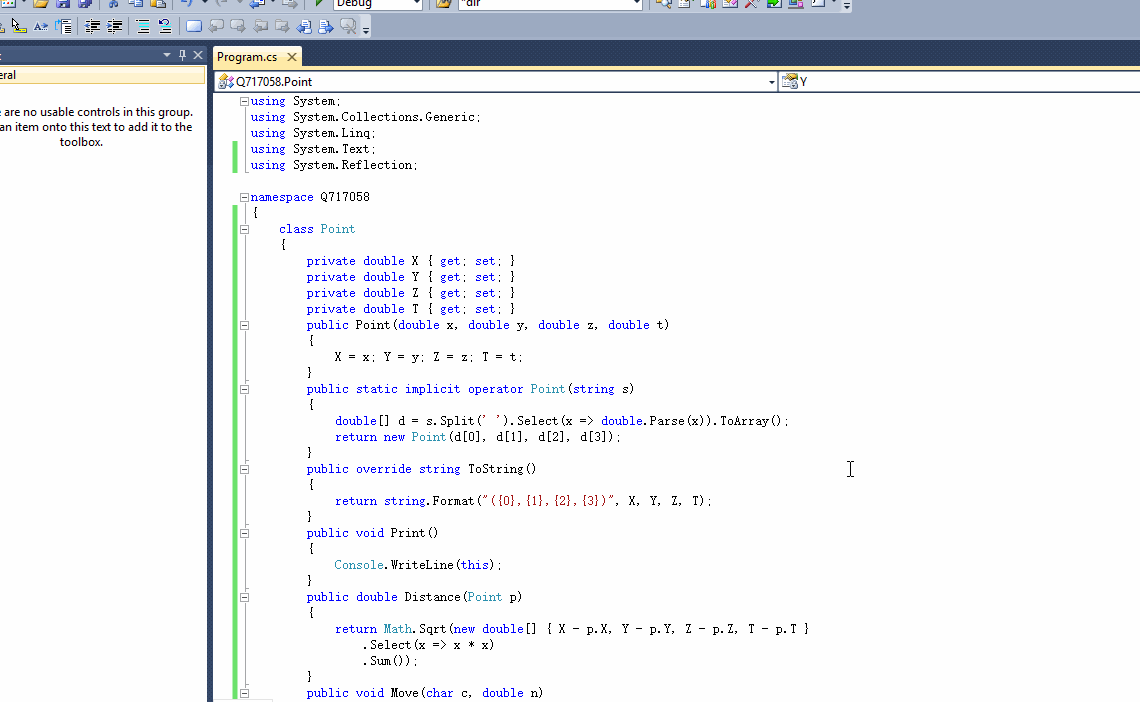2018-12-14 08:46

# C#控制台程序，面向对象的问题请看图片• 写回答
• 关注问题
• 收藏
• 邀请回答

#### 1条回答默认 最新

•threenewbee 2018-12-14 11:43
已采纳
``````using System;
using System.Collections.Generic;
using System.Linq;
using System.Text;
using System.Reflection;

namespace Q717058
{
class Point
{
private double X { get; set; }
private double Y { get; set; }
private double Z { get; set; }
private double T { get; set; }
public Point(double x, double y, double z, double t)
{
X = x; Y = y; Z = z; T = t;
}
public static implicit operator Point(string s)
{
double[] d = s.Split(' ').Select(x => double.Parse(x)).ToArray();
return new Point(d, d, d, d);
}
public override string ToString()
{
return string.Format("({0},{1},{2},{3})", X, Y, Z, T);
}
public void Print()
{
Console.WriteLine(this);
}
public double Distance(Point p)
{
return Math.Sqrt(new double[] { X - p.X, Y - p.Y, Z - p.Z, T - p.T }
.Select(x => x * x)
.Sum());
}
public void Move(char c, double n)
{
var prop = GetType().GetProperty(c.ToString().ToUpper(), BindingFlags.NonPublic | BindingFlags.Instance);
if (prop != null)
prop.SetValue(this, (double)prop.GetValue(this, null) + n, null);
}
}
class Program
{
static void Main(string[] args)
{